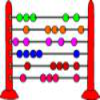#### You may also like### Which Scripts?

There are six numbers written in five different scripts. Can you sort out which is which?### A Romantic Riddle

Read this riddle and see if you can work out how the trees must be planted.### Fibonacci's Three Wishes 1

First of two articles about Fibonacci, written for students.

# Roman Numerals

##### Age 7 to 11Challenge Level
These symbols are the building blocks of Roman numerals:

I, V, X, L, C, D and M

Do you know the value of each letter?  Click on 'Show' to check...

I = 1
V = 5
X = 10
L = 50
C = 100
D = 500
M = 1000

In our number system (the Arabic numeral system), there are ten different digits, (0, 1, 2 , 3, 4, 5, 6, 7, 8, 9) and the place of these digits in the number determines its value. For example, 2 on its own means 'two', but in 3240, the '2' now means "two hundred". In this way, any number can be written down, using only ten digits.

Roman numerals have a set of rules which allow you to write down any number:

1. If a smaller numeral comes after a larger numeral, add the smaller number to the larger number;
2. If a smaller numeral comes before a larger numeral, subtract the smaller number from the larger number;
3. Do not use the same symbol more than three times in a row.

Can you use these rules to construct and decipher Roman numerals?
Try converting the following Roman numerals into Arabic numerals:

III
IV
XVIII
XIX
MCMLXXVI
MMXXIII
MCMLXII

Now try converting the following into Roman numerals:

55
86
two thousand five hundred and ninety-two
913

Can you work out how to write '1984' in Roman numerals?

'1984' written in Roman numerals has more numerals than when it is written in Arabic numerals. However, this will not always be the case.

Can you find some examples when the number of Roman numerals is fewer than the number of Arabic numerals for the same number?